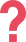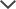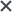I got the ACCUPLACER pack. I found TestPrep-Online’s preparation pack very helpful. The questions and study guides are great. I found that the explanations and solving tips really helped me to learn the material and improve my score.
Nicole
I got the TEAS tests. I found this website to be very helpful. The practice tests are almost identical to the real test; they have helped me a lot in studying.
Chelsea
I used the GMAT prep. It's almost standing in one line with formal GMAT simulations.
Daniel
I used the LSAT practice material. It helped improve my technique a lot.
Steve
We used the NYC G&T pack. We ordered the materials a week before the test. My son was very happy and felt confident. Thanks!
Malak
We got the CogAT tests for my daughter. I loved how quickly I was able to access the tests after purchasing the product and how easy it was to use! It familiarized my daughter with the format of the test, allowing her to feel calm for the test.
Margaret

This page provides a breakdown of the score ranges for the three ACCUPLACER math assessments. Use the quick links below to navigate the page.

## ACCUPLACER Arithmetic Scores

The arithmetic section of the ACCUPLACER exam consists of 17 questions which determine your knowledge in three areas of basic arithmetic:

• whole numbers and fractions
• decimals and percentages
• applications

## ACCUPLACER Arithmetic Score Range

Your ACCUPLACER Arithmetic score places you in one of five skills groups:

20-37 38-64 65-92 93-109 110
Low
Arithmetic Skills
Minimal Arithmetic Skills Basic Arithmetic Skills Sufficient Arithmetic Skills Substantial Arithmetic Skills

For a greater breakdown of the skill groups, you must either be able to apply or have mastered the skills listed in each category below.

### Low Arithmetic Skills

Students can perform the following tasks:

• Calculate an average, given integer values
• Identify data represented by simple graphs
• Make conversions between fractions, decimals, and percents
• Perform the basic arithmetic operations of addition, subtraction, multiplication, and division using whole numbers, fractions, decimals, and mixed numbers
• Estimate products and squares of decimals, and square roots of whole numbers and decimals
• Divide whole numbers by decimals and fractions
• Solve simple word problems involving fractions, ratio, percent increase and decrease, and area
• Solve simple percent problems of the form p% of q = ? and ?% of q = r

### Minimal Arithmetic Skills

Students have mastered the skills in the Low Arithmetic score group. Students are able to do the following:

• Find equivalent forms of fractions
• Estimate calculations involving fractions

### Basic Arithmetic Skills

Students have mastered the skills in the Minimal Arithmetic and Low Arithmetic score groups. Students are able to perform the following tasks:

• Solve word problems involving the manipulation of units of measurement
• Solve complex word problems involving percent, average, and proportional reasoning
• Find the square root of decimal numbers
• Solve simple number sentences involving a variable

### Sufficient Arithmetic Skills

Students have mastered the skills in the Basic Arithmetic, Minimal Arithmetic, and Low Arithmetic score groups. Students can apply learned knowledge and solve problems from all skills groups with ease.

### Substantial Arithmetic Skills

Students who have mastered all skills groups and consistently deliver high scores in all tests may receive a score that places them in the Substantial Arithmetic Skills group.

## ACCUPLACER Elementary Algebra Scores

The elementary algebra portion of the ACCUPLACER exam consists of 12 questions that determine your knowledge in three areas of elementary algebra:

• integers and rationals
• algebraic expressions
• equations, inequalities, and word problems
20-43 44-81 82-108 109
Minimal
Elementary Algebra Skills
Basic
Elementary Algebra Skills
Sufficient
Elementary Algebra Skills
Substantial
Elementary Algebra Skills

For a more substantial break down of the score groups, read below to learn about the skills that students must master or at least be able to apply.

### Minimal Elementary Algebra Skills

Students can perform the following tasks:

• Perform operations with signed numbers
• Combine like terms
• Multiply binomials
• Evaluate algebraic expressions
• Factor the difference of squares
• Square binomials
• Solve linear equations with integer coefficients

### Basic Elementary Algebra Skills

Students have mastered the Minimal Algebra score group skillset and can complete the following tasks:

• Solve linear equations with integer coefficients
• Simplify algebraic expressions

### Sufficient Elementary Algebra Skills

Students have mastered the skill sets for both the Minimal Algebra and Basic Algebra score groups, and can perform the following tasks:

• Simplify algebraic expressions
• Factor quadratic expressions where a=1
• Solve linear equations with fractional and literal coefficients, and linear inequalities with integer coefficients
• Solve systems of equations
• Identify graphical properties of equations and inequalities

### Substantial Elementary Algebra Skills

Students who have mastered all score group skill sets for Elementary Algebra and can consistently apply learned knowledge and perform well in ACCUPLACER Elementary Algebra testing may score within the Substantial Elementary Algebra Skills score group.

## Increase Your ACCUPLACER Math Score

Want to improve your score? Need help with some of these mathematical concepts? Access over 1,000 ACCUPLACER questions with answer explanations to guide you through the rules and processes required for high scores in Elementary Algebra testing.

## ACCUPLACER College-Level Math Scores

The Elementary Algebra section of the ACCUPLACER exam consists of 20 questions which determine your knowledge in six areas:

• algebraic operations
• solutions of equations and inequalities
• coordinate geometry
• applications and other algebra topics
• functions
• trigonometry
20-39 40-62 63-85 86-102 103
Low
College-Level Math Skills
Minimal
College-Level Math Skills
Basic
College-Level Math Skills
Sufficient
College-Level Math Skills
Substantial
College-Level Math Skills

Students must be able to apply or in some cases master a particular set of skills. For a more substantial break down of the score groups, read below to learn about the skills that students must master or at least be able to apply.

### Low College-Level Math Skills

Students can complete the following tasks:

• Identify common factors
• Factor binomials and trinomials
• Manipulate factors to simplify complex fractions
• Simplify and perform arithmetic operations with rational expressions, including complex fractions
• Work with algebraic expressions involving real number exponents
• Factor polynomial expressions
• Solve and graph linear equations and inequalities
• Solve quadratic equations by factoring
• Solve absolute value equations
• Graph simple parabolas
• Determine the value of a function for a specific number in the domain
• Determine the value of the composition of two functions
• Understand basic geometry and trigonometry

### Minimal College-Level Math Skills

Students have mastered the Low College-Level Math skill set and can perform the following tasks:

• Evaluate and simplify expressions involving functional notation, including composition of functions
• Understand polynomial functions
• Solve simple equations involving trigonometric functions, logarithmic functions, and exponential functions

### Basic College-Level Math Skills

Students have mastered the Low College-Level Math and Minimal College-Level Math skill sets, and can complete the following tasks:

• Perform algebraic operations and solve equations with complex numbers
• Solve right-triangle problems
• Understand trigonometric functions and their inverses
• Solve trigonometric equations
• Manipulate trigonometric identities
• Understand the relationship between exponents and logarithms
• Understand the rules that govern the manipulation of exponents and logarithms
• Recognize graphic properties of functions such as absolute value, quadratic, and logarithmic

### Sufficient College-Level Math Skills

Students have mastered the Low College-Level Math, Minimal College-Level Math, and Basic College-Level Math skill sets.

### Substantial College-Level Math Skills

Students who have mastered all the skill sets for the College-Level Math scoring groups and who deliver strong performances during testing sessions may achieve a score that places them in the Substantial College-Level Math Skills score group.

## Improve Your ACCUPLACER Math Score!

For ACCUPLACER math practice questions, start preparing now with our ACCUPLACER Math Pack. If you would like practice for both math and English sections of the ACCUPLACER test, we offer a comprehensive package of over 1,000 questions, with answers and explanations, available in our ACCUPLACER Premium Pack.

ACCUPLACER® and WritePlacer® are trademarks and property of COLLEGE BOARD. The trademark holder is not affiliated with TestPrep-Online or this website.

Need HelpNeed Help